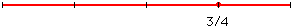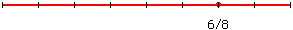Name: D. F. Level of question: elementary Who is asking: Student Question: What is the mathematical reason equivalent fractions are equal? Hi, Equivalent fractions are "equal" because they represent the same quantity. For example suppose that you take a line segment and divide it into four equal parts then the length of three of these parts is three-quarters of the length of the line segment.If, however, you divide the line segment into eight equal parts then the length of six of these parts is six-eights of the length of the line segment.But these two division points are at exactly the same place so the lengths are equal. Thus 3/4 the length of the line segment has a length equal to 6/8 of the length of the line segment. Hence we say that 3/4 and 6/8 are equal. This is a definition or explaination of what the word equal means when applied to fractions. Two fractions are equal if they represent the same proportion of the whole. 3/4 = 6/8 since three-quarters of a whole is exacly the same quantity as 6/8 of a whole. Cheers, Penny Go to Math Central